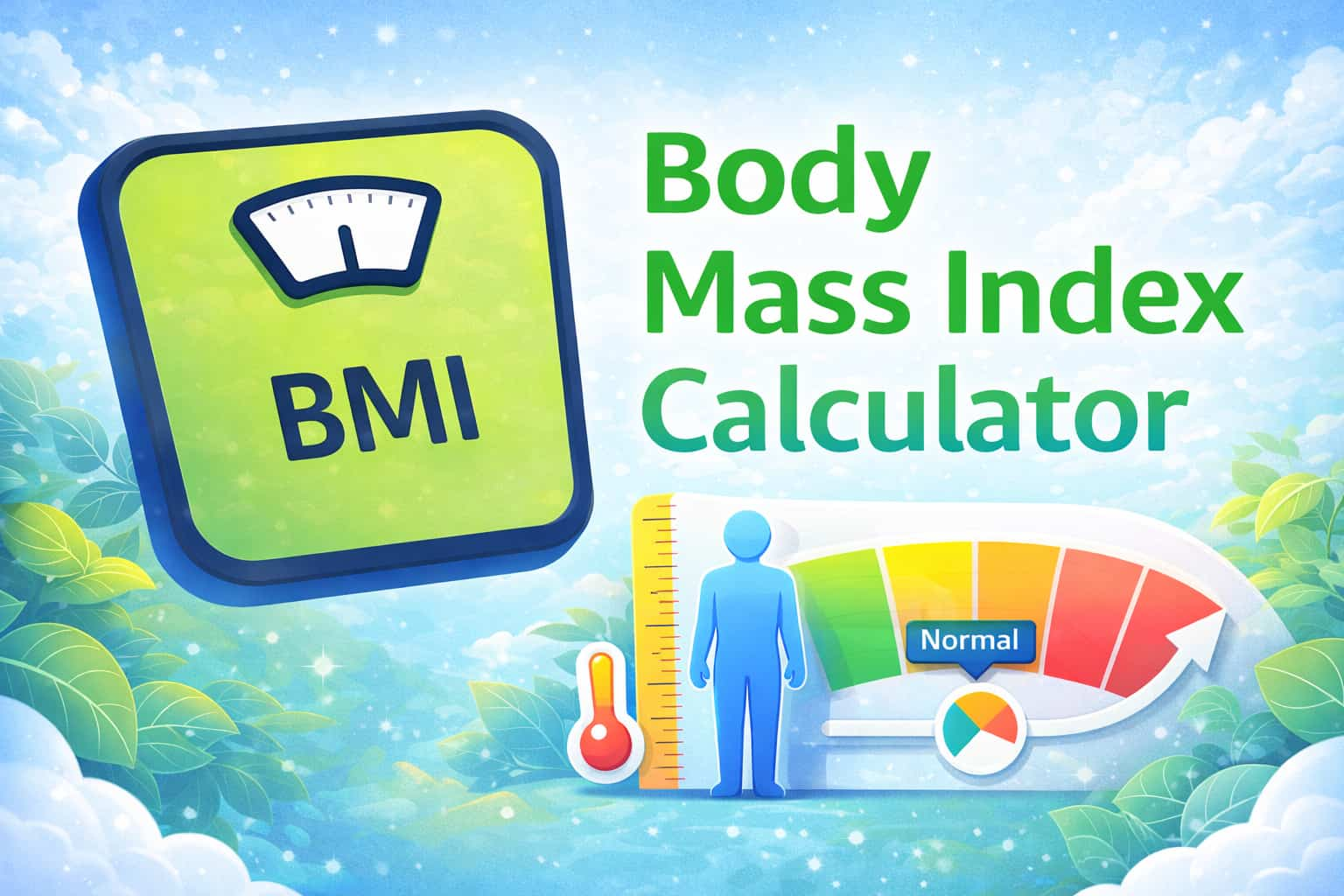## Wednesday, October 16, 2019

### Body Mass Index Calculations in R

One exercise I get students to do in one of my early R Programming classes is write code to calculate Body Mass Index (BMI), and then to use an if statement to determine what the value of the BMI indicates about your health. BMI is a gross estimate for the amount of fat in your body - the categories are as follows:Image source: JoyDeepDev.

BMI is calculated as: BMI = Weight (kg) / Height (m) x Height (m). Before my recent (medically advised) diet, my weight was 104 kgs. My height is 1.82 metres - my BMI is therefore 31.4, which put me firmly into the "Obese" category. This is a term which I would not have referred to myself as although I am heavy - I didn't consider myself "obese".

Here is the R code that I use in class:

#
# Short Exercise - calculate BMI
#